# Backtest with Brinson Attribution to Evaluate Portfolio Performance

This example shows how to compute Brinson attribution using the output of the MATLAB® backtest framework. The backtest framework allows you to build custom trading strategies and then backtest them against historical or simulated market data. The example uses the Brinson attribution function (`brinsonAttribution`) to explain a portfolio's performance versus a benchmark.

Brinson attribution requires that a category is assigned to each asset. Load the `dowPortfolio.xlsx` data set, then assign each of the 30 stocks to a category.

```% Read a table of daily adjusted close prices for 2006 DJIA stocks. pricesTT = readtimetable('dowPortfolio.xlsx'); % Remove the index from the dataset. pricesTT= removevars(pricesTT,'DJI'); num_assets = width(pricesTT); % Set the sectors for each stock. asset_categories = categorical(["Materials";"Financials";"Financials"; ... "Industrials";"Financials";"Industrials";"Materials"; ... "Communication Services";"Industrials";"Consumer Discretionary"; ... "Consumer Discretionary";"Industrials";"Information Technology"; ... "Information Technology";"Information Technology";"Health Care"; ... "Financials";"Consumer Staples";"Consumer Discretionary"; ... "Industrials";"Consumer Staples";"Health Care"; ... "Information Technology";"Health Care";"Consumer Staples"; ... "Communication Services";"Industrials";"Communication Services"; ... "Consumer Staples";"Energy"]); % Display the stock categories. Symbol = pricesTT.Properties.VariableNames(:); table(Symbol,asset_categories)```
```ans=30×2 table Symbol asset_categories ________ ______________________ {'AA' } Materials {'AIG' } Financials {'AXP' } Financials {'BA' } Industrials {'C' } Financials {'CAT' } Industrials {'DD' } Materials {'DIS' } Communication Services {'GE' } Industrials {'GM' } Consumer Discretionary {'HD' } Consumer Discretionary {'HON' } Industrials {'HPQ' } Information Technology {'IBM' } Information Technology {'INTC'} Information Technology {'JNJ' } Health Care ⋮ ```

### Define and Create Backtest Strategies

Multiperiod Brinson attribution expects fixed, regular time periods. In this case, the backtest strategy rebalance schedules will match the Brinson periods. You can set the rebalance dates to be monthly starting from January first.

```% Rebalance on the first trading day of each month. rebalance_schedule = datetime(2006,1,1):calmonths(1):datetime(2006,12,1); for idx = 1:numel(rebalance_schedule) priceIdx = find(rebalance_schedule(idx) <= pricesTT.Dates,1,'first'); rebalance_schedule(idx) = pricesTT.Dates(priceIdx); end```

The risk budgeting strategy needs historical data in order to set initial weights. You can use the first month of price data as a warm-up period to set the initial weights. The backtest begins at the end of the warm-up period.

```% Use first month as warm-up period for the risk budgeting strategy. start_date = rebalance_schedule(2); % Get the first month of prices to initialize the risk budgeting strategy. initial_prices = pricesTT(pricesTT.Dates <= start_date,:);```

Brinson attribution measures portfolio manager performance against some benchmark. For this example, use a simple equal-weighted strategy as the benchmark. This example examines the performance of the following two strategies relative to this benchmark:

• Equal Category — This strategy allocates resources equally per category, and then allocates equal weights for stocks within each category.

• Risk Budgeting — This strategy sets weights for stocks with the goal of minimizing each asset's risk contribution.

Create a `backtestStrategy` object for the benchmark and the two candidate strategies. Define each of these strategies to use the same rebalance schedule and assign the appropriate initial weights.

```% Define the Benchmark strategy. benchmark = backtestStrategy('Benchmark',@equalWeightRebalanceFcn, ... 'RebalanceFrequency',rebalance_schedule, ... 'InitialWeights',computeEqualWeights(num_assets), ... 'UserData',struct()); % Define the equal category strategy. equalCategory = backtestStrategy('EqualCategory',@equalCategoryRebalanceFcn, ... 'RebalanceFrequency',rebalance_schedule, ... 'InitialWeights',computeEqualCategory(asset_categories), ... 'UserData',struct('Categories',asset_categories)); % Define the risk budgeting strategy. riskBudgeting = backtestStrategy('RiskBudgeting',@riskBudgetingRebalanceFcn, ... 'RebalanceFrequency',rebalance_schedule, ... 'InitialWeights',computeRiskBudgeting(initial_prices));```

Use `backtestEngine` to create a `backtestEngine` object for the strategies and then use `runBacktest` to run the backtest. Display the equity curve using `equityCurve` and then use `summary` to display the backtest summary table. Both the equal category and risk budgeting strategies outperform the benchmark.

```strategies = [benchmark,equalCategory,riskBudgeting]; bt = backtestEngine(strategies); bt = runBacktest(bt,pricesTT,'Start',start_date); equityCurve(bt)```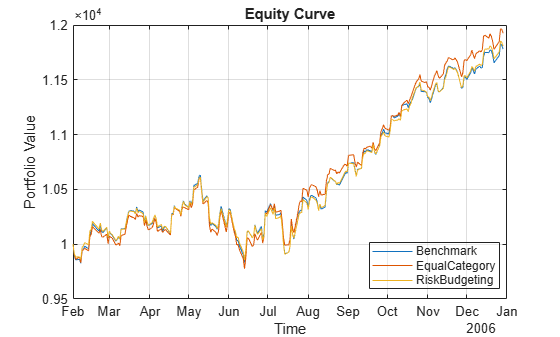`summary(bt)`
```ans=9×3 table Benchmark EqualCategory RiskBudgeting __________ _____________ _____________ TotalReturn 0.17811 0.19249 0.18065 SharpeRatio 0.11671 0.12645 0.12393 Volatility 0.0062905 0.0062211 0.0059849 AverageTurnover 0.00073556 0.00076049 0.0015965 MaxTurnover 0.025681 0.028611 0.080538 AverageReturn 0.00073259 0.00078493 0.00074008 MaxDrawdown 0.07502 0.078323 0.071364 AverageBuyCost 0 0 0 AverageSellCost 0 0 0 ```

### Prepare Backtest Results for Brinson Attribution

Set the strategy name of the benchmark.

`benchmark_name = 'Benchmark';`

Define Brinson periods by the rebalance dates and the backtest end date.

`period_dates = [rebalance_schedule(2:end), pricesTT.Dates(end)];`

### Compute Brinson Attribution for Backtested Investment Strategy

Select whether to analyze the equal category or risk budgeting strategy. Then, use the `backtest2brinson` function in Local Functions to compute `asset_table` that is formatted for use with the `brinsonAttribution` function. For more information on the format of the `asset_table`, see the description for the `AssetTable` input argument in the `brinsonAttribution` function.

```% Select strategy to analyze strategy_name =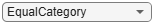"EqualCategory"; asset_table = backtest2brinson(bt,strategy_name,benchmark_name,pricesTT,asset_categories,period_dates); head(asset_table)```
``` Period Name Return Category PortfolioWeight BenchmarkWeight ______ _____ _________ ______________________ _______________ _______________ 1 "AA" -0.050283 Materials 0.055556 0.033333 1 "AIG" 0.0015356 Financials 0.027778 0.033333 1 "AXP" 0.028439 Financials 0.027778 0.033333 1 "BA" 0.022929 Industrials 0.018519 0.033333 1 "C" 0.015045 Financials 0.027778 0.033333 1 "CAT" 0.074416 Industrials 0.018519 0.033333 1 "DD" 0.037791 Materials 0.055556 0.033333 1 "DIS" 0.11182 Communication Services 0.037037 0.033333 ```
```% Compute Brinson attribution brinson = brinsonAttribution(asset_table); summary(brinson)```
```ans=11×1 table Brinson Attribution Summary ___________________________ Total Number of Assets 30 Number of Assets in Portfolio 30 Number of Assets in Benchmark 30 Number of Periods 11 Number of Categories 9 Portfolio Return 0.19249 Benchmark Return 0.17811 Active Return 0.014376 Allocation Effect 0.014376 Selection Effect 8.5567e-18 Interaction Effect -1.1701e-19 ```

Generate the attribution chart using `attributionsChart` with the `brinson` object. The attributions chart creates a horizontal bar chart of portfolio performance attributions by category, aggregated over all time periods.

`attributionsChart(brinson)`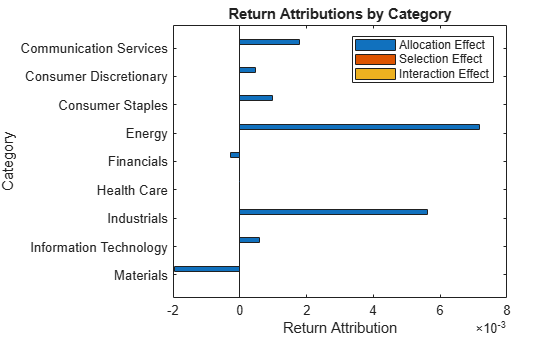Generate a category returns chart using `categoryReturnsChart` with the `brinson` object. The category returns chart creates a horizontal bar chart of portfolio and benchmark category returns, aggregated over all time periods.

`categoryReturnsChart(brinson)`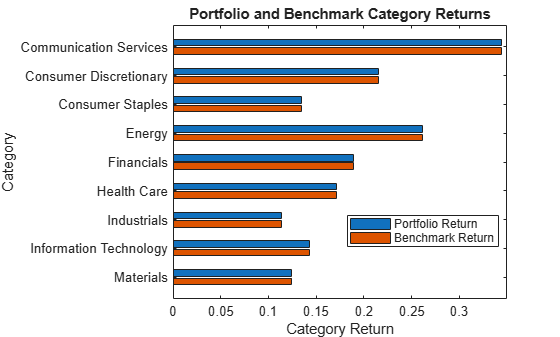Generate a category weights chart using `categoryWeightsChart` with the `brinson` object. Te category weights chart creates a horizontal bar chart of portfolio, benchmark, and active weights by category, averaged over all time periods.

`categoryWeightsChart(brinson)`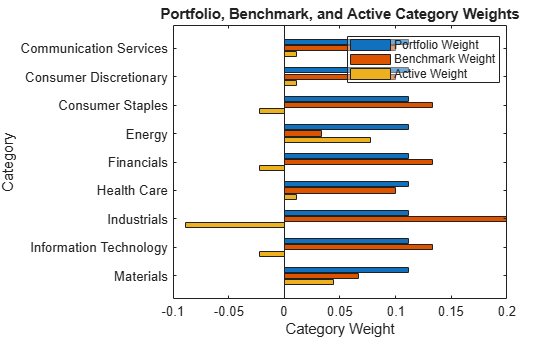The equal category strategy, despite having different category weights from the benchmark, has the same equal-weighted asset selection process within each category. Therefore, the equal category strategy has no selection or interaction effect (relative to the benchmark). Meanwhile, the risk budgeting strategy disregards the asset categories when setting portfolio weights, so it has selection and interaction effects.

### Local Functions

Both the equal asset weight and equal category weight rebalance functions compute a fixed weight that is assigned at each rebalance date. In order to avoid calculating the weights each time, the weights are calculated once and then the result is saved in the `UserData` struct for the `backtestStrategy` object. For more information on the `UserData` struct, see the rebalanceFcn input argument for `backtestStrategy`.

```function [new_weights,user_data] = equalWeightRebalanceFcn(~,pricesTT,user_data) % Equal asset weight rebalance function if ~isfield(user_data,'FixedAllocation') % If this is the first call to the rebalance function, calculate the % desired fixed allocation and save it. user_data.FixedAllocation = computeEqualWeights(width(pricesTT)); end new_weights = user_data.FixedAllocation; end function weights = computeEqualWeights(num_assets) % Equal asset weight portfolio allocation weights = ones(1,num_assets) / num_assets; end function [new_weights,user_data] = equalCategoryRebalanceFcn(~, ~, user_data) % Equal category weight rebalance function if ~isfield(user_data,'FixedAllocation') % If this is the first call to the rebalance function, calculate the % desired fixed allocation and save it. user_data.FixedAllocation = computeEqualCategory(user_data.Categories); end new_weights = user_data.FixedAllocation; end function weights = computeEqualCategory(asset_categories) % Equal category weight portfolio allocation weights = zeros(1,numel(asset_categories)); unique_categories = unique(asset_categories); category_weight = 1 / numel(unique_categories); for i = 1:numel(unique_categories) category_mask = asset_categories == unique_categories(i); weights(category_mask) = category_weight / sum(category_mask); end end function new_weights = riskBudgetingRebalanceFcn(~,pricesTT) % Risk budgeting rebalance function new_weights = computeRiskBudgeting(pricesTT); end function new_weights = computeRiskBudgeting(pricesTT) % Risk budgeting portfolio allocation asset_returns = tick2ret(pricesTT); asset_cov = cov(asset_returns{:,:}); new_weights = riskBudgetingPortfolio(asset_cov); end function asset_table = backtest2brinson(bt,strategy_name,benchmark_name,pricesTT,asset_categories,period_dates) % Build Brinson attribution input asset table based on the results of the % completed backtest. % Compute asset returns per period asset_returns = tick2ret(pricesTT(period_dates,:)); num_periods = height(asset_returns); % Brinson Return input Return = asset_returns.Variables'; % Brinson Category input Category = repmat(asset_categories,1,num_periods); % Brinson Period input num_assets = width(pricesTT); Period = (1:num_periods) .* ones(num_assets,1); % Brinson Name input Name = repmat(string(pricesTT.Properties.VariableNames(:)),1,num_periods); % Benchmark weights benchmark_positions = bt.Positions.(benchmark_name){period_dates(1:end-1),2:end}'; BenchmarkWeight = benchmark_positions ./ sum(benchmark_positions); % Strategy weights portfolio_positions = bt.Positions.(strategy_name){period_dates(1:end-1),2:end}'; PortfolioWeight = portfolio_positions ./ sum(portfolio_positions); % Aggregate the inputs into the Brinson asset table asset_table = table(Period(:),Name(:),Return(:),Category(:), ... PortfolioWeight(:),BenchmarkWeight(:),... VariableNames=["Period","Name","Return","Category", ... "PortfolioWeight","BenchmarkWeight"]); end```